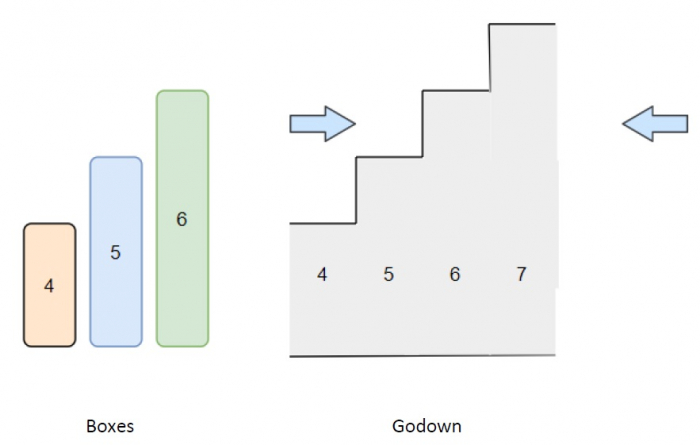# Program to find out how many boxes can be put into the godown in Python

Suppose, we have two arrays containing integers. One list contains the height of some unit width boxes and another array contains the height of rooms in the godown. The rooms are numbered 0...n, and the height of the rooms is provided in their respective indexes in the array godown. We have to find out the number of boxes that can be pushed into the godown. a few things have to be kept in mind,

• The boxes can’t be put one on another.

• The order of the boxes can be changed.

The boxes are put into the godown from any side, it can either be from the left or the right. If a box is taller than the height of the room, then the box along with all the boxes to its right cannot be pushed into the godown.

So, if the input is like boxes = [4, 5, 6], godown = [4, 5, 6, 7], then the output will be 3 All three boxes given as input can be put into the godown.To solve this, we will follow these steps −

• sort the list boxes in a descending order

• l := 0

• r := size of godown - 1

• bi := 0

• ret := 0

• while bi < size of boxes and l <= r, do

• if godown[l] > godown[r], then

• if boxes[bi] <= godown[l], then

• ret := ret + 1

• l := l + 1

• otherwise,

• if boxes[bi] <= godown[r], then

• ret := ret + 1

• r := r - 1

• bi := bi + 1

• return ret

## Example (Python)

Let us see the following implementation to get better understanding −

Live Demo

def solve(boxes, godown):
boxes.sort(reverse = True)

l, r = 0, len(godown) - 1
bi, ret = 0, 0
while bi < len(boxes) and l <= r:
if godown[l] > godown[r]:
if boxes[bi] <= godown[l]:
ret += 1
l += 1
else:
if boxes[bi] <= godown[r]:
ret += 1
r -= 1
bi += 1

return ret

print(solve([4, 5, 6], [4, 5, 6, 7]))

## Input

[4, 5, 6], [4, 5, 6, 7]

## Output

3